Скачать презентацию V O P YJS Speech production mechanisms Y J

00002f846250212fa699d649e24f14a1.ppt

• Количество слайдов: 54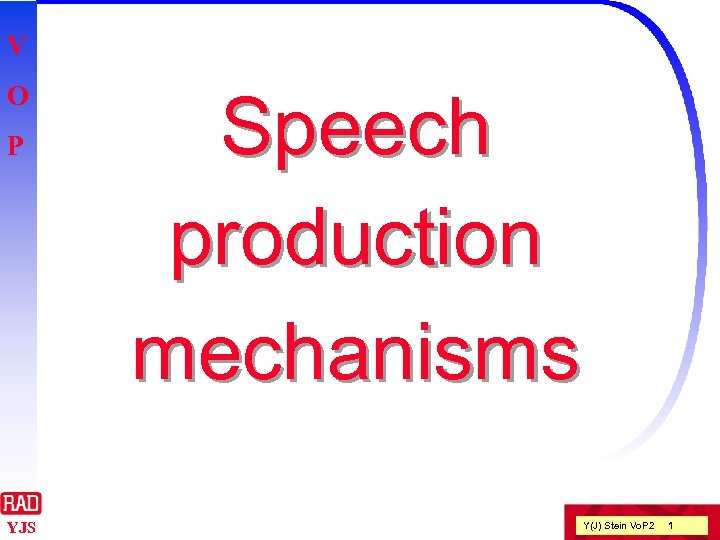V O P YJS Speech production mechanisms Y(J) Stein Vo. P 2 1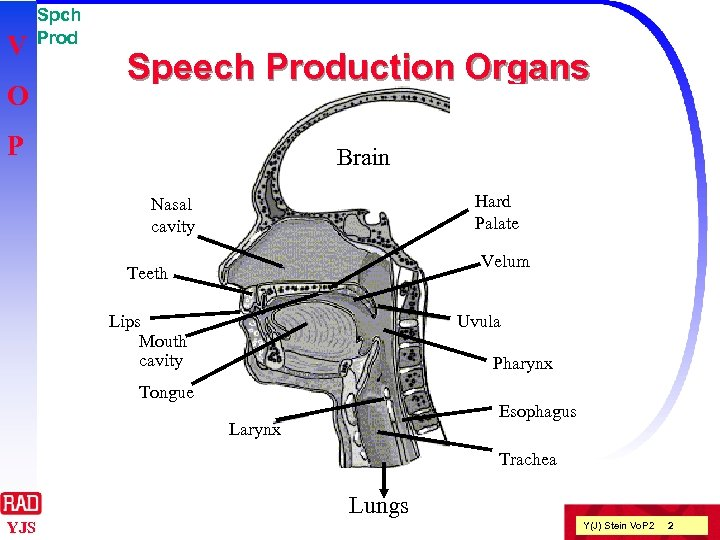V O Spch Prod Speech Production Organs P Brain Hard Palate Nasal cavity Velum Teeth Lips Mouth cavity Uvula Pharynx Tongue Esophagus Larynx Trachea Lungs YJS Y(J) Stein Vo. P 2 2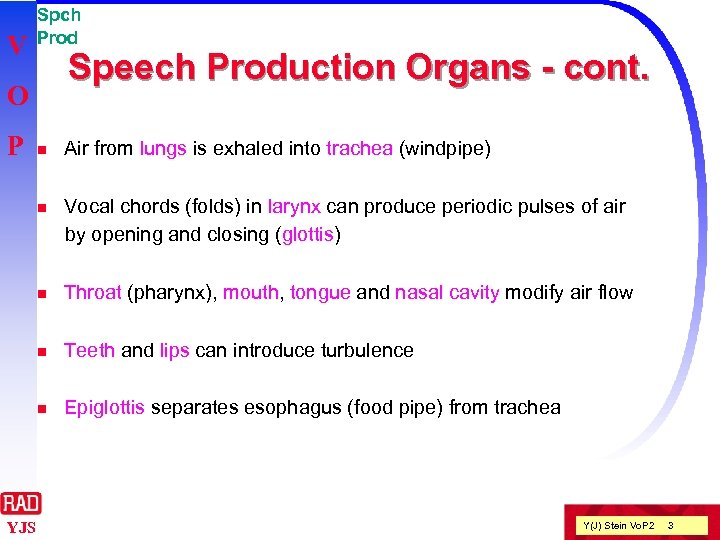V Spch Prod Speech Production Organs - cont. O P Air from lungs is exhaled into trachea (windpipe) n Vocal chords (folds) in larynx can produce periodic pulses of air by opening and closing (glottis) n Throat (pharynx), mouth, tongue and nasal cavity modify air flow n Teeth and lips can introduce turbulence n YJS n Epiglottis separates esophagus (food pipe) from trachea Y(J) Stein Vo. P 2 3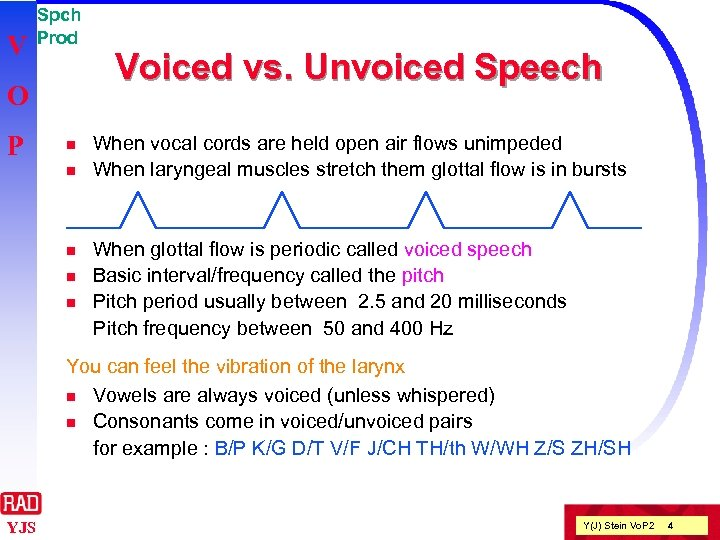V Spch Prod O P n n n Voiced vs. Unvoiced Speech When vocal cords are held open air flows unimpeded When laryngeal muscles stretch them glottal flow is in bursts When glottal flow is periodic called voiced speech Basic interval/frequency called the pitch Pitch period usually between 2. 5 and 20 milliseconds Pitch frequency between 50 and 400 Hz You can feel the vibration of the larynx n Vowels are always voiced (unless whispered) n Consonants come in voiced/unvoiced pairs for example : B/P K/G D/T V/F J/CH TH/th W/WH Z/S ZH/SH YJS Y(J) Stein Vo. P 2 4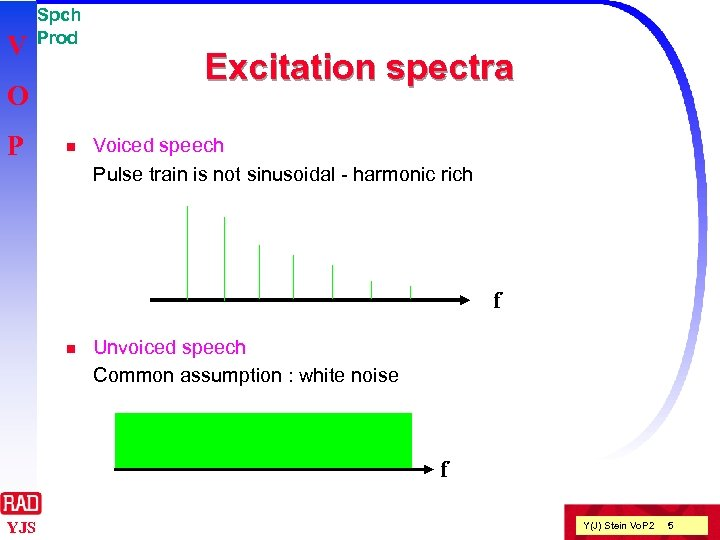V Spch Prod O P n Excitation spectra Voiced speech Pulse train is not sinusoidal - harmonic rich f n Unvoiced speech Common assumption : white noise f YJS Y(J) Stein Vo. P 2 5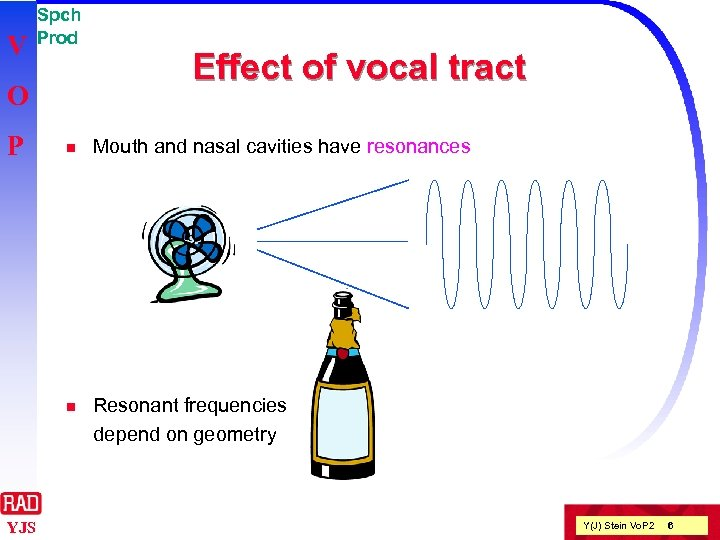V Spch Prod O P Effect of vocal tract Mouth and nasal cavities have resonances n YJS n Resonant frequencies depend on geometry Y(J) Stein Vo. P 2 6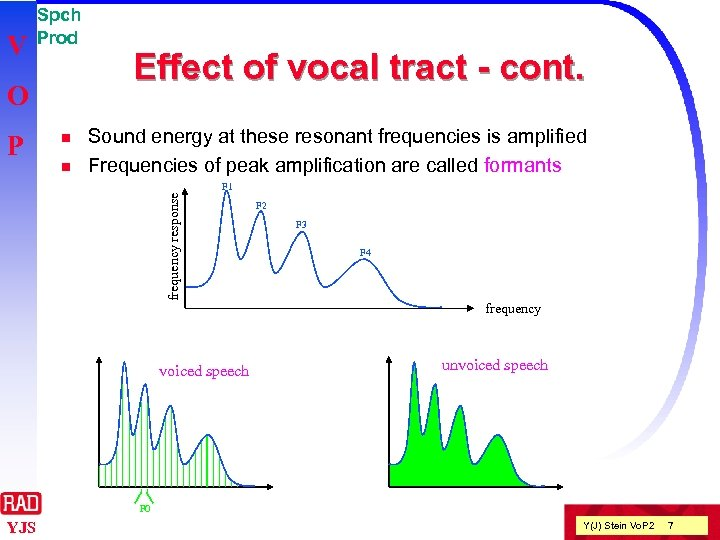V Spch Prod O P n n Effect of vocal tract - cont. Sound energy at these resonant frequencies is amplified Frequencies of peak amplification are called formants frequency response F 1 voiced speech F 2 F 3 F 4 frequency unvoiced speech F 0 YJS Y(J) Stein Vo. P 2 7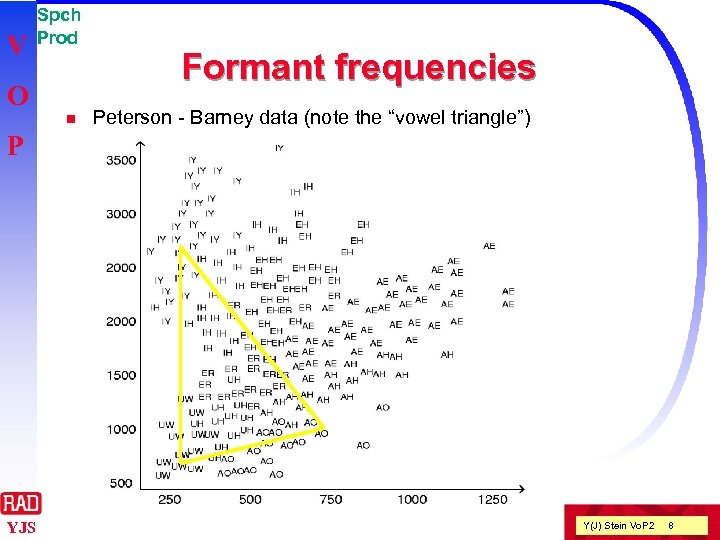V Spch Prod O n Formant frequencies Peterson - Barney data (note the “vowel triangle”) P YJS Y(J) Stein Vo. P 2 8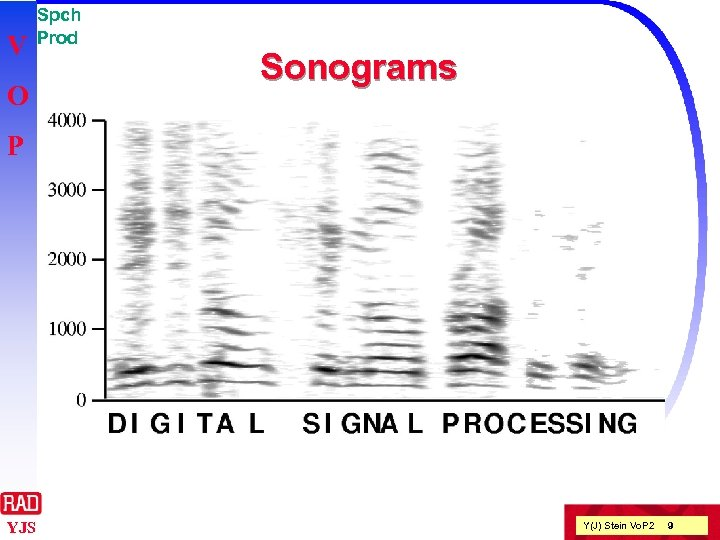V O Spch Prod Sonograms P YJS Y(J) Stein Vo. P 2 9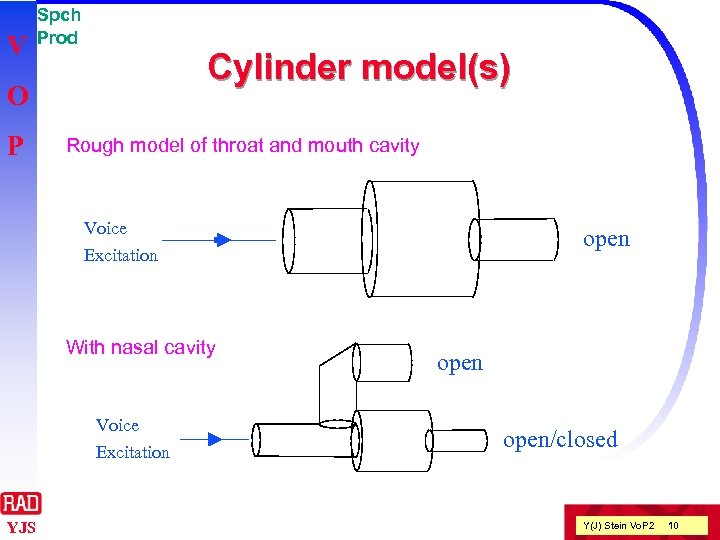V Spch Prod Cylinder model(s) O P Rough model of throat and mouth cavity Voice open Excitation With nasal cavity Voice Excitation YJS open/closed Y(J) Stein Vo. P 2 10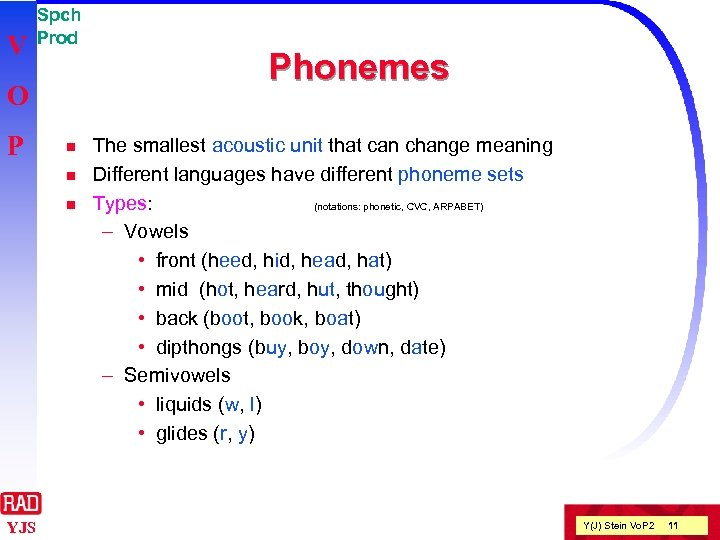V Spch Prod O P n n n YJS Phonemes The smallest acoustic unit that can change meaning Different languages have different phoneme sets Types: (notations: phonetic, CVC, ARPABET) – Vowels • front (heed, hid, head, hat) • mid (hot, heard, hut, thought) • back (boot, book, boat) • dipthongs (buy, boy, down, date) – Semivowels • liquids (w, l) • glides (r, y) Y(J) Stein Vo. P 2 11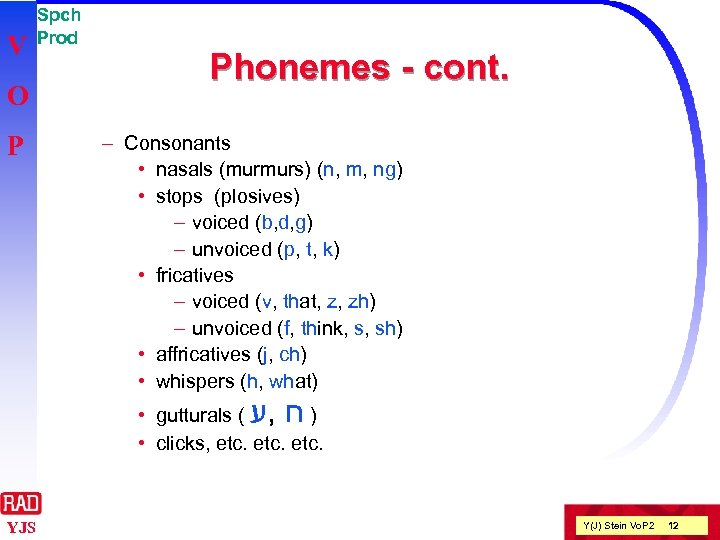V O P Spch Prod Phonemes - cont. – Consonants • nasals (murmurs) (n, m, ng) • stops (plosives) – voiced (b, d, g) – unvoiced (p, t, k) • fricatives – voiced (v, that, z, zh) – unvoiced (f, think, s, sh) • affricatives (j, ch) • whispers (h, what) • gutturals ( ) ח , ע • clicks, etc. YJS Y(J) Stein Vo. P 2 12V Spch Prod Basic LPC Model O P Pulse Generator U/V Switch LPC synthesis filter White Noise Generator YJS Y(J) Stein Vo. P 2 13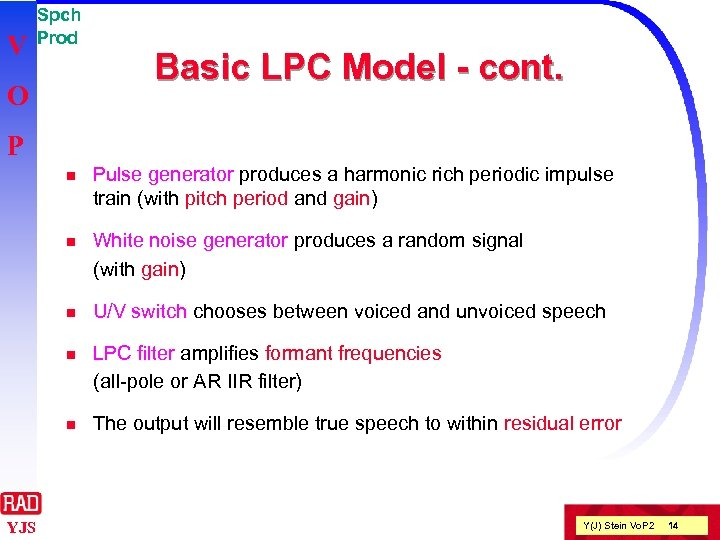V Spch Prod O Basic LPC Model - cont. P n n White noise generator produces a random signal (with gain) n U/V switch chooses between voiced and unvoiced speech n LPC filter amplifies formant frequencies (all-pole or AR IIR filter) n YJS Pulse generator produces a harmonic rich periodic impulse train (with pitch period and gain) The output will resemble true speech to within residual error Y(J) Stein Vo. P 2 14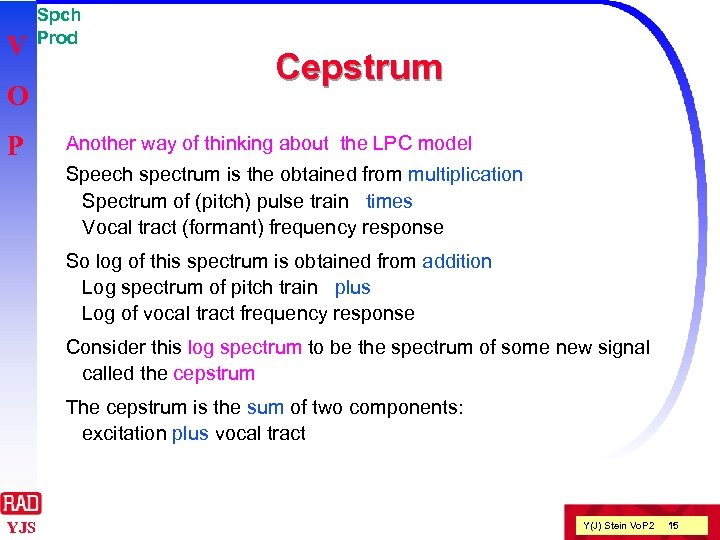V O P Spch Prod Cepstrum Another way of thinking about the LPC model Speech spectrum is the obtained from multiplication Spectrum of (pitch) pulse train times Vocal tract (formant) frequency response So log of this spectrum is obtained from addition Log spectrum of pitch train plus Log of vocal tract frequency response Consider this log spectrum to be the spectrum of some new signal called the cepstrum The cepstrum is the sum of two components: excitation plus vocal tract YJS Y(J) Stein Vo. P 2 15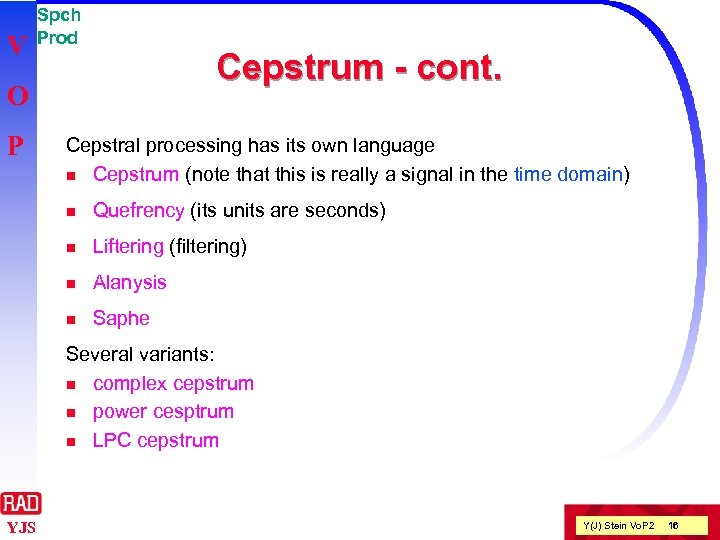V Spch Prod Cepstrum - cont. O P Cepstral processing has its own language n Cepstrum (note that this is really a signal in the time domain) n Quefrency (its units are seconds) n Liftering (filtering) n Alanysis n Saphe Several variants: n complex cepstrum n power cesptrum n LPC cepstrum YJS Y(J) Stein Vo. P 2 16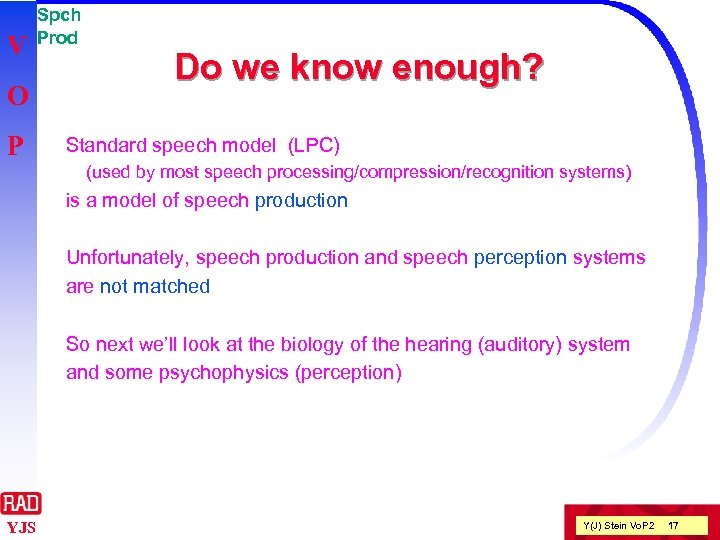V O P Spch Prod Do we know enough? Standard speech model (LPC) (used by most speech processing/compression/recognition systems) is a model of speech production Unfortunately, speech production and speech perception systems are not matched So next we’ll look at the biology of the hearing (auditory) system and some psychophysics (perception) YJS Y(J) Stein Vo. P 2 17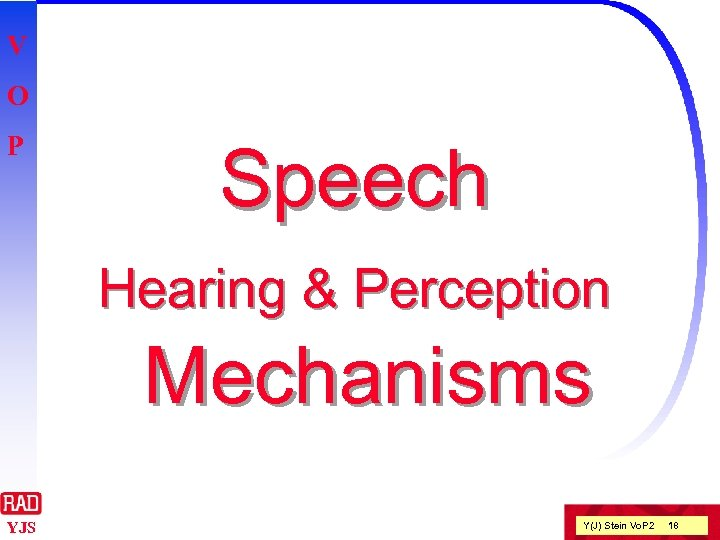V O P Speech Hearing & Perception Mechanisms YJS Y(J) Stein Vo. P 2 18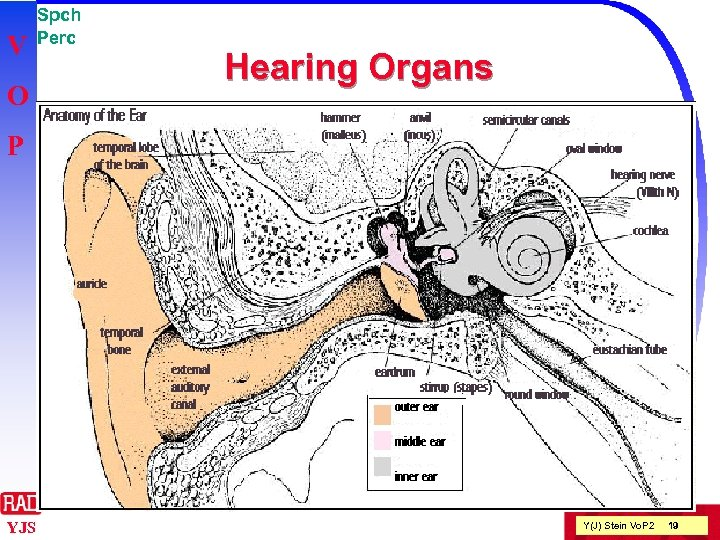V O Spch Perc Hearing Organs P YJS Y(J) Stein Vo. P 2 19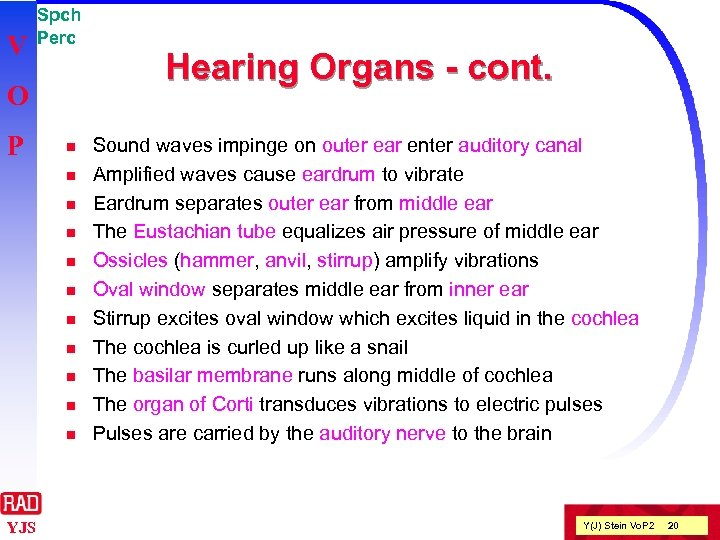V Spch Perc O P n n n YJS Hearing Organs - cont. Sound waves impinge on outer ear enter auditory canal Amplified waves cause eardrum to vibrate Eardrum separates outer ear from middle ear The Eustachian tube equalizes air pressure of middle ear Ossicles (hammer, anvil, stirrup) amplify vibrations Oval window separates middle ear from inner ear Stirrup excites oval window which excites liquid in the cochlea The cochlea is curled up like a snail The basilar membrane runs along middle of cochlea The organ of Corti transduces vibrations to electric pulses Pulses are carried by the auditory nerve to the brain Y(J) Stein Vo. P 2 20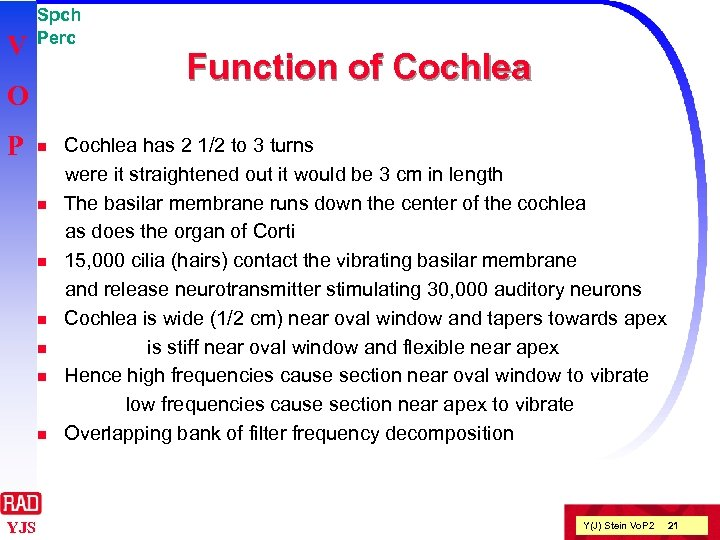V Spch Perc O P n n n n YJS Function of Cochlea has 2 1/2 to 3 turns were it straightened out it would be 3 cm in length The basilar membrane runs down the center of the cochlea as does the organ of Corti 15, 000 cilia (hairs) contact the vibrating basilar membrane and release neurotransmitter stimulating 30, 000 auditory neurons Cochlea is wide (1/2 cm) near oval window and tapers towards apex is stiff near oval window and flexible near apex Hence high frequencies cause section near oval window to vibrate low frequencies cause section near apex to vibrate Overlapping bank of filter frequency decomposition Y(J) Stein Vo. P 2 21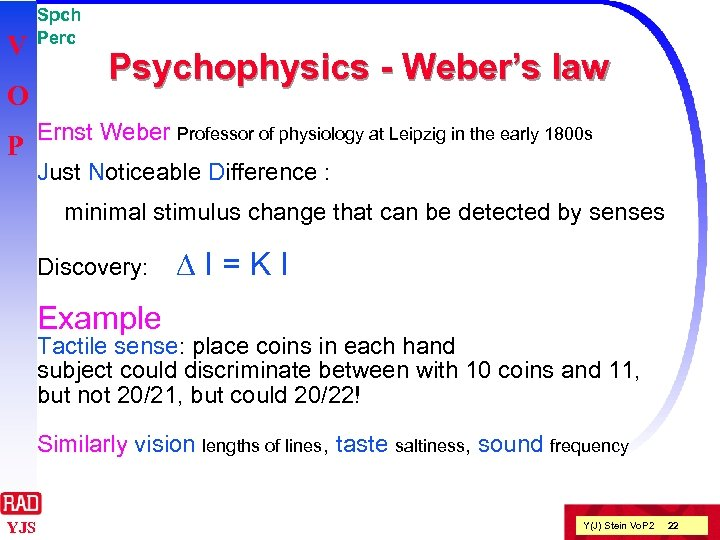V O P Spch Perc Psychophysics - Weber’s law Ernst Weber Professor of physiology at Leipzig in the early 1800 s Just Noticeable Difference : minimal stimulus change that can be detected by senses Discovery: DI=KI Example Tactile sense: place coins in each hand subject could discriminate between with 10 coins and 11, but not 20/21, but could 20/22! Similarly vision lengths of lines, taste saltiness, sound frequency YJS Y(J) Stein Vo. P 2 22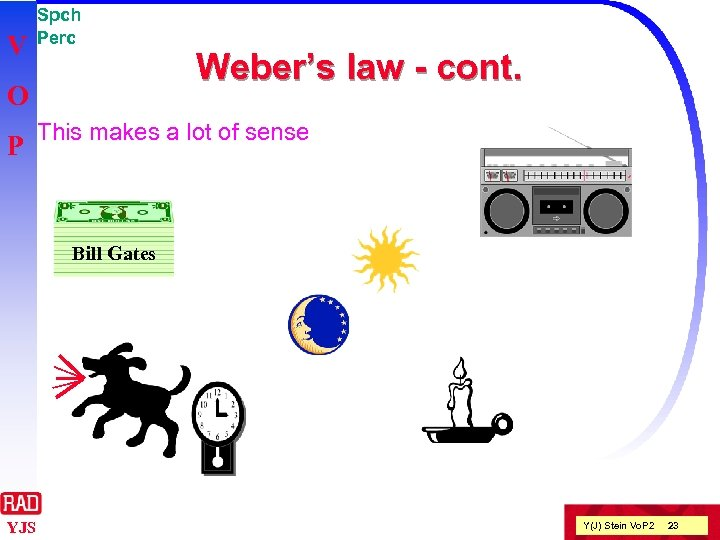V Spch Perc O P Weber’s law - cont. This makes a lot of sense Bill Gates YJS Y(J) Stein Vo. P 2 23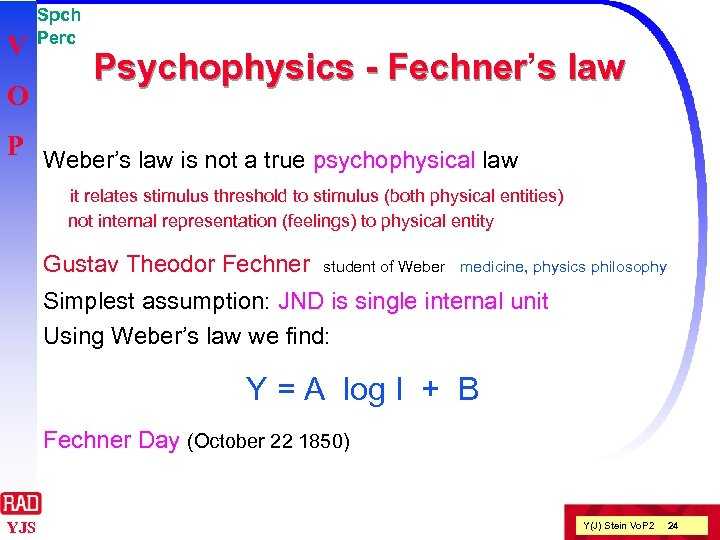V O Spch Perc Psychophysics - Fechner’s law Fechner P Weber’s law is not a true psychophysical law it relates stimulus threshold to stimulus (both physical entities) not internal representation (feelings) to physical entity Gustav Theodor Fechner student of Weber medicine, physics philosophy Simplest assumption: JND is single internal unit Using Weber’s law we find: Y = A log I + B Fechner Day (October 22 1850) YJS Y(J) Stein Vo. P 2 24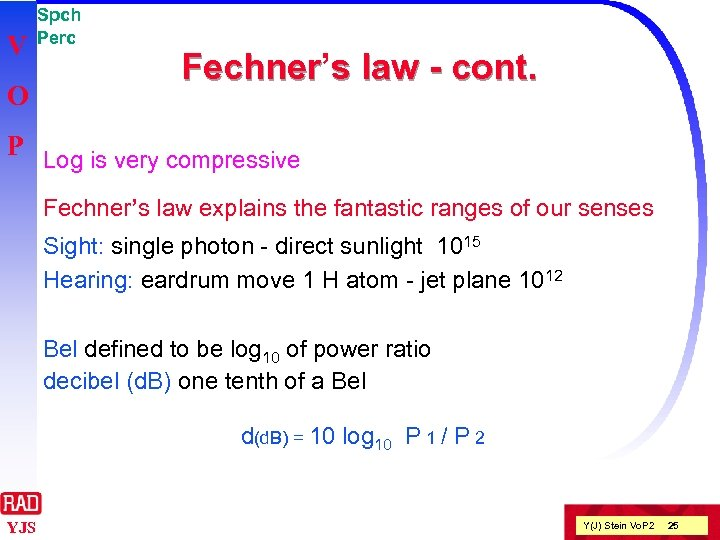V O Spch Perc Fechner’s law - cont. Fechner P Log is very compressive Fechner’s law explains the fantastic ranges of our senses Sight: single photon - direct sunlight 1015 Hearing: eardrum move 1 H atom - jet plane 1012 Bel defined to be log 10 of power ratio decibel (d. B) one tenth of a Bel d(d. B) = 10 log 10 P 1 / P 2 YJS Y(J) Stein Vo. P 2 25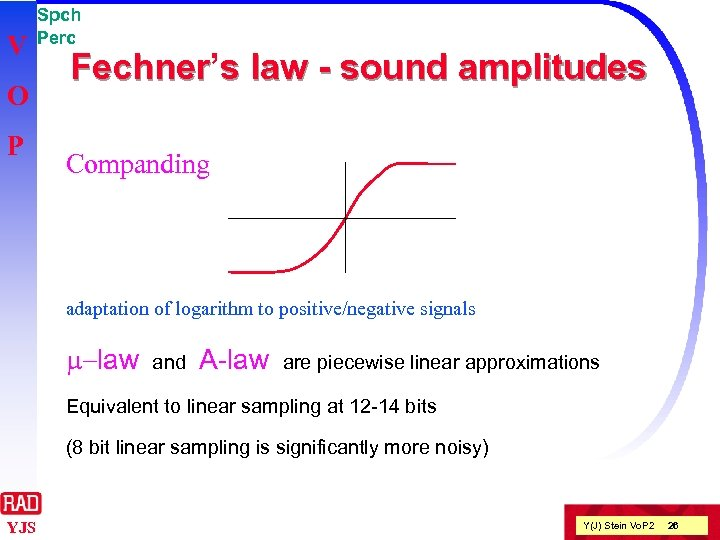V O P Spch Perc Fechner’s law - sound amplitudes Fechner Companding adaptation of logarithm to positive/negative signals m-law and A-law are piecewise linear approximations Equivalent to linear sampling at 12 -14 bits (8 bit linear sampling is significantly more noisy) YJS Y(J) Stein Vo. P 2 26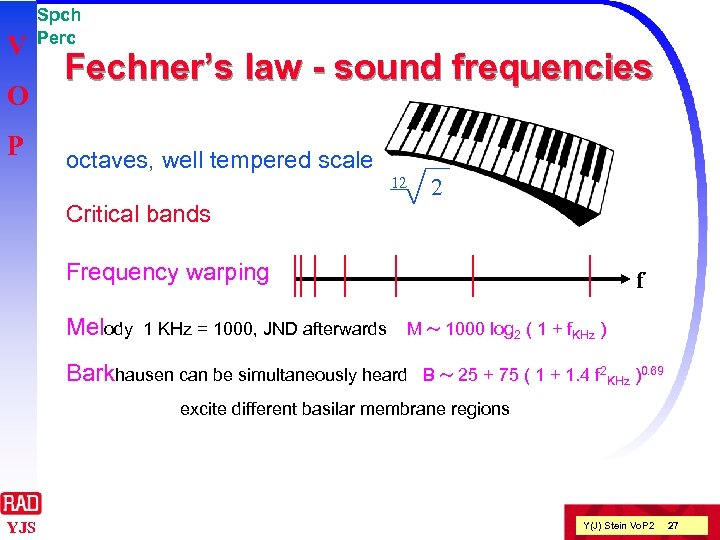V O P Spch Perc Fechner’s law - sound frequencies octaves, well tempered scale 12 2 Critical bands Frequency warping Melody 1 KHz = 1000, JND afterwards f M ~ 1000 log 2 ( 1 + f. KHz ) Barkhausen can be simultaneously heard B ~ 25 + 75 ( 1 + 1. 4 f 2 KHz )0. 69 excite different basilar membrane regions YJS Y(J) Stein Vo. P 2 27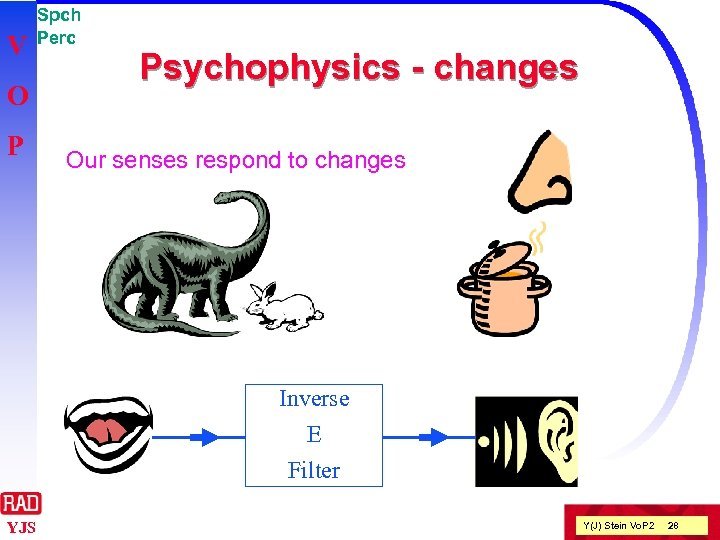V O P Spch Perc Psychophysics - changes Our senses respond to changes Inverse E Filter YJS Y(J) Stein Vo. P 2 28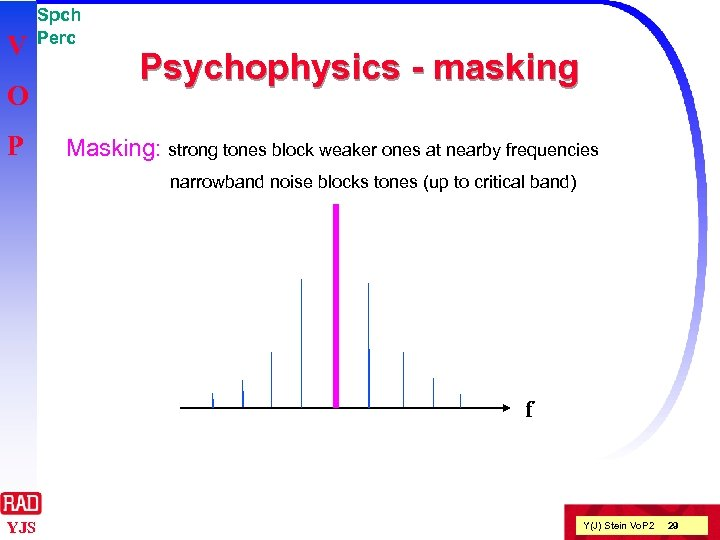V O P Spch Perc Psychophysics - masking Masking: strong tones block weaker ones at nearby frequencies narrowband noise blocks tones (up to critical band) f YJS Y(J) Stein Vo. P 2 29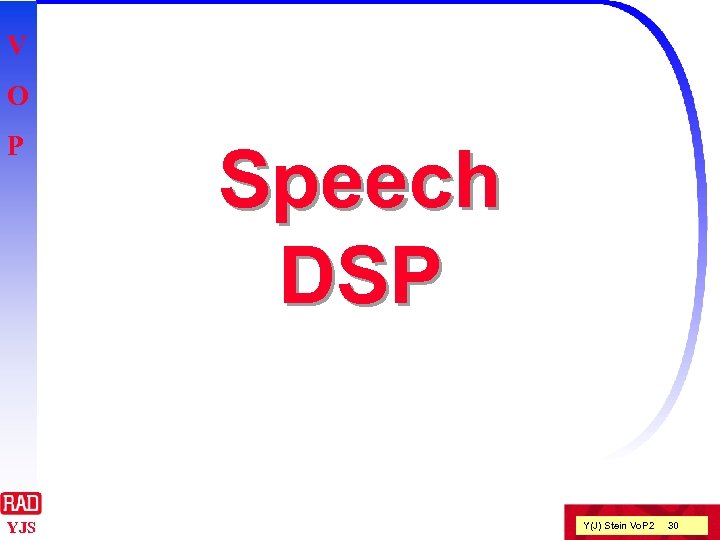V O P YJS Speech DSP Y(J) Stein Vo. P 2 30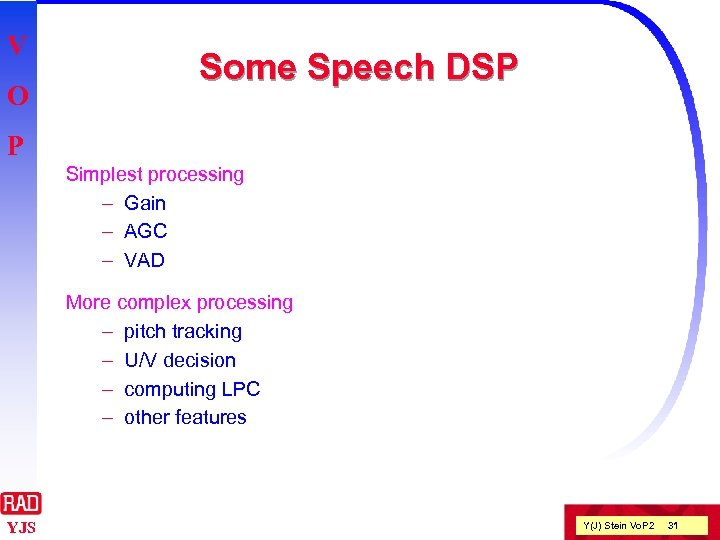V O Some Speech DSP P Simplest processing – Gain – AGC – VAD More complex processing – pitch tracking – U/V decision – computing LPC – other features YJS Y(J) Stein Vo. P 2 31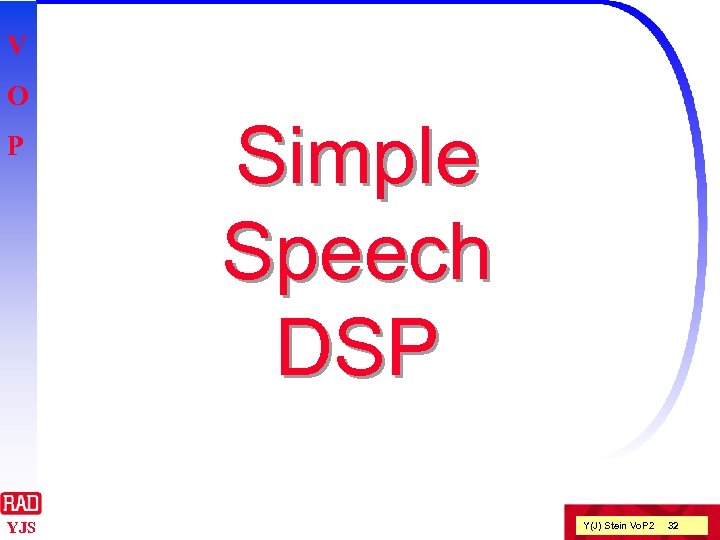V O P YJS Simple Speech DSP Y(J) Stein Vo. P 2 32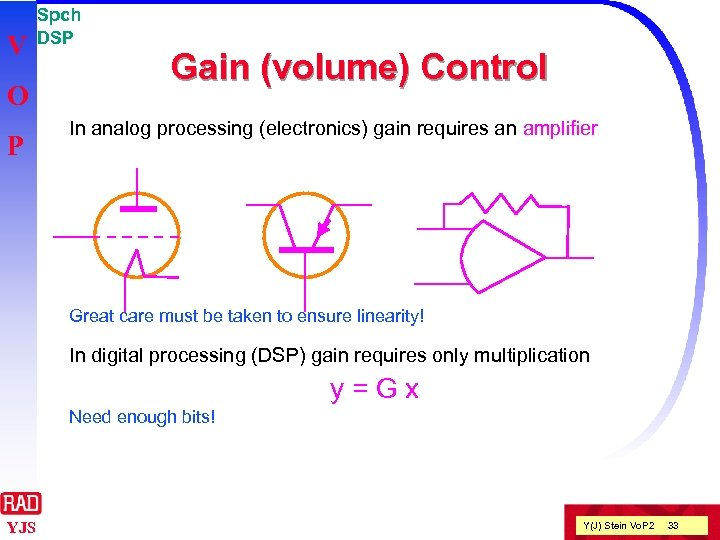V O P Spch DSP Gain (volume) Control In analog processing (electronics) gain requires an amplifier Great care must be taken to ensure linearity! In digital processing (DSP) gain requires only multiplication y=Gx Need enough bits! YJS Y(J) Stein Vo. P 2 33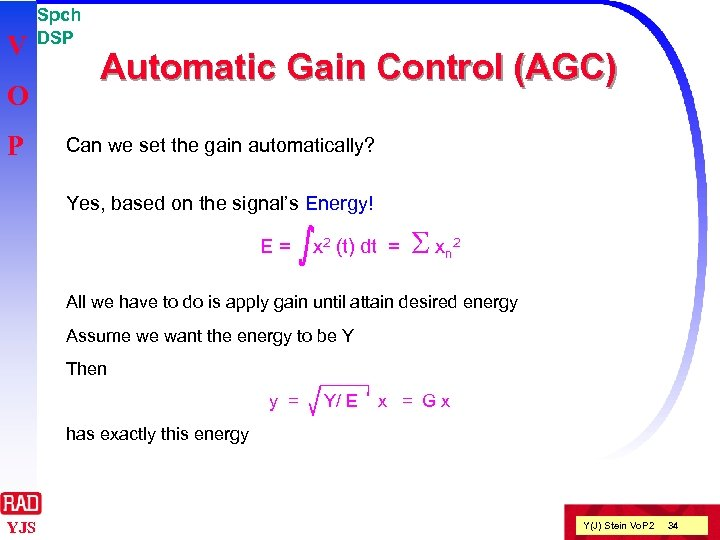V O P Spch DSP Automatic Gain Control (AGC) Can we set the gain automatically? Yes, based on the signal’s Energy! E= x 2 (t) dt = S xn 2 All we have to do is apply gain until attain desired energy Assume we want the energy to be Y Then y = Y/ E x = Gx has exactly this energy YJS Y(J) Stein Vo. P 2 34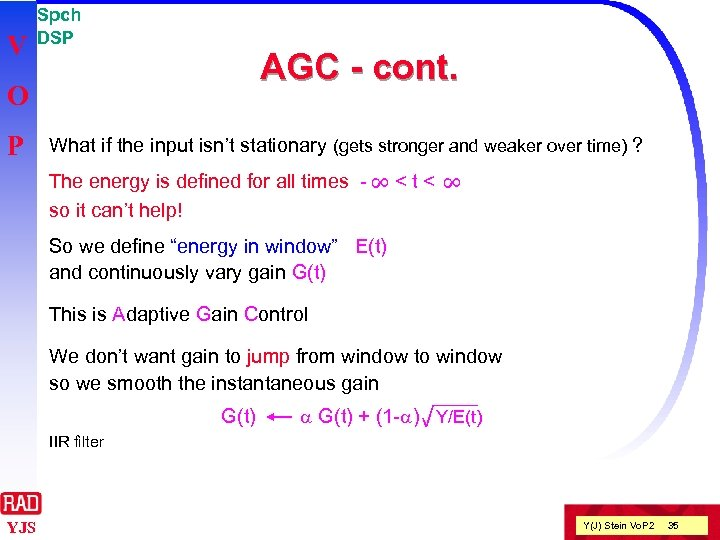V Spch DSP AGC - cont. O P What if the input isn’t stationary (gets stronger and weaker over time) ?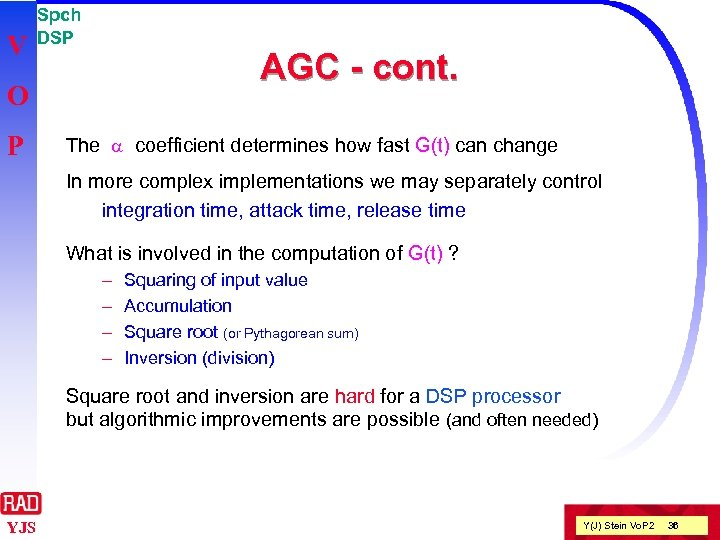V Spch DSP AGC - cont. O P The a coefficient determines how fast G(t) can change In more complex implementations we may separately control integration time, attack time, release time What is involved in the computation of G(t) ? – – Squaring of input value Accumulation Square root (or Pythagorean sum) Inversion (division) Square root and inversion are hard for a DSP processor but algorithmic improvements are possible (and often needed) YJS Y(J) Stein Vo. P 2 36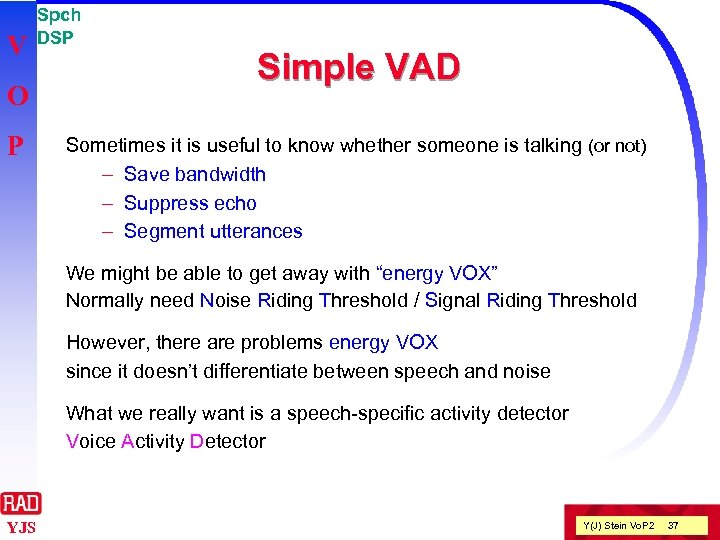V O P Spch DSP Simple VAD Sometimes it is useful to know whether someone is talking (or not) – Save bandwidth – Suppress echo – Segment utterances We might be able to get away with “energy VOX” Normally need Noise Riding Threshold / Signal Riding Threshold However, there are problems energy VOX since it doesn’t differentiate between speech and noise What we really want is a speech-specific activity detector Voice Activity Detector YJS Y(J) Stein Vo. P 2 37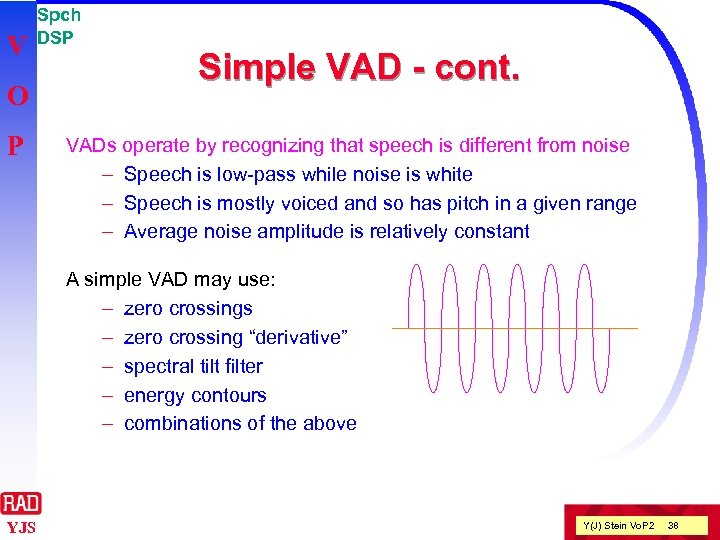V O P Spch DSP Simple VAD - cont. VADs operate by recognizing that speech is different from noise – Speech is low-pass while noise is white – Speech is mostly voiced and so has pitch in a given range – Average noise amplitude is relatively constant A simple VAD may use: – zero crossings – zero crossing “derivative” – spectral tilt filter – energy contours – combinations of the above YJS Y(J) Stein Vo. P 2 38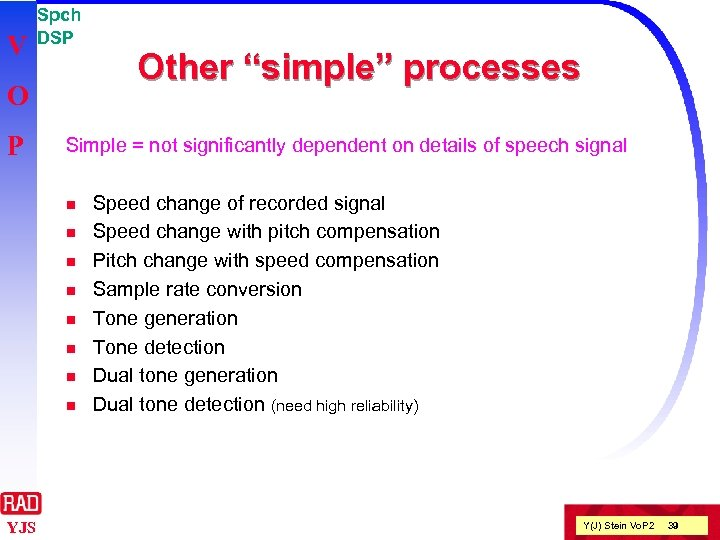V Spch DSP O P Simple = not significantly dependent on details of speech signal n n n n YJS Other “simple” processes Speed change of recorded signal Speed change with pitch compensation Pitch change with speed compensation Sample rate conversion Tone generation Tone detection Dual tone generation Dual tone detection (need high reliability) Y(J) Stein Vo. P 2 39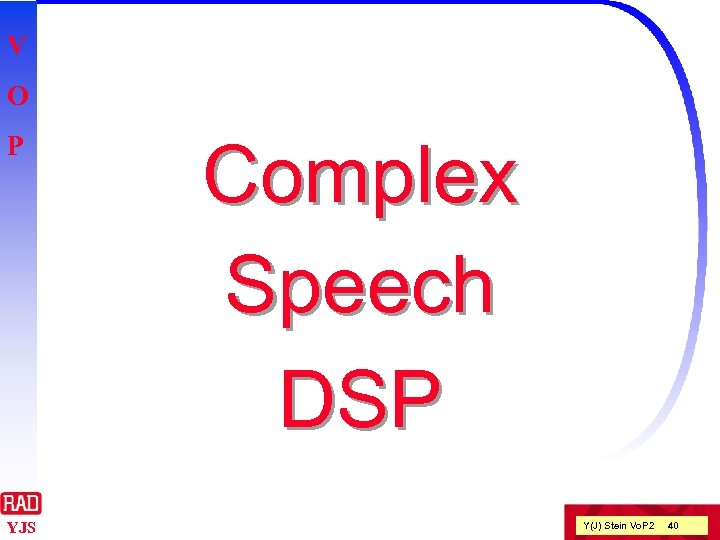V O P YJS Complex Speech DSP Y(J) Stein Vo. P 2 40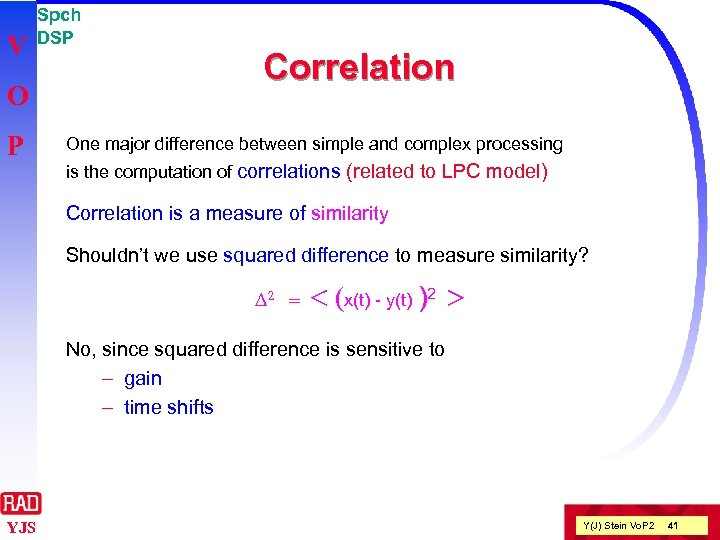V O P Spch DSP Correlation One major difference between simple and complex processing is the computation of correlations (related to LPC model) Correlation is a measure of similarity Shouldn’t we use squared difference to measure similarity? D 2 = < (x(t) - y(t) )2 > No, since squared difference is sensitive to – gain – time shifts YJS Y(J) Stein Vo. P 2 41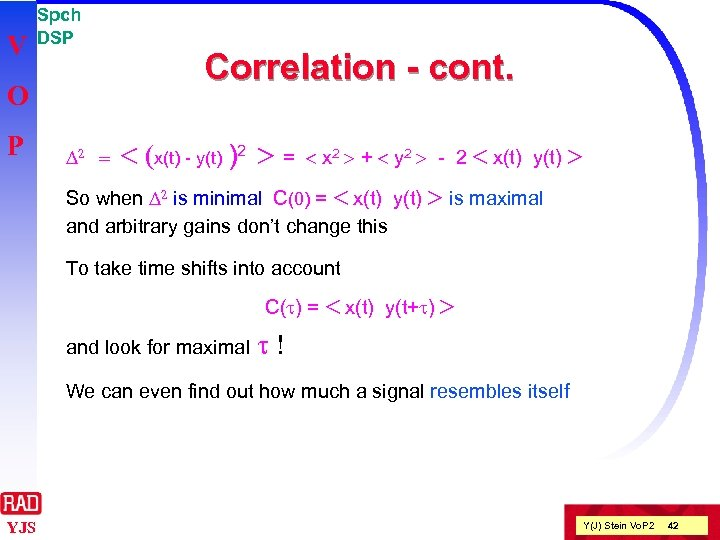V Spch DSP O P D 2 = Correlation - cont. < (x(t) - y(t) )2 > = < x 2 > + < y 2 > - 2 < x(t) y(t) > So when D 2 is minimal C(0) = < x(t) y(t) > is maximal and arbitrary gains don’t change this To take time shifts into account C(t) = < x(t) y(t+t) > and look for maximal t! We can even find out how much a signal resembles itself YJS Y(J) Stein Vo. P 2 42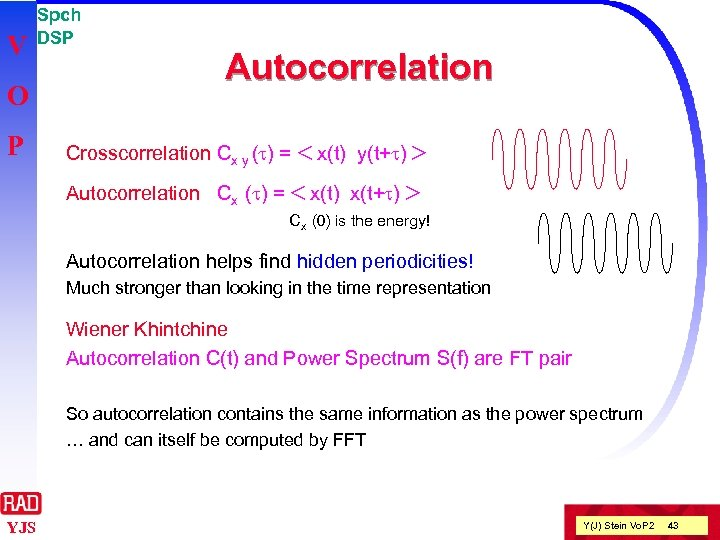V O P Spch DSP Autocorrelation Crosscorrelation Cx y (t) = < x(t) y(t+t) > Autocorrelation Cx (t) = < x(t) x(t+t) > Cx (0) is the energy! Autocorrelation helps find hidden periodicities! Much stronger than looking in the time representation Wiener Khintchine Autocorrelation C(t) and Power Spectrum S(f) are FT pair So autocorrelation contains the same information as the power spectrum … and can itself be computed by FFT YJS Y(J) Stein Vo. P 2 43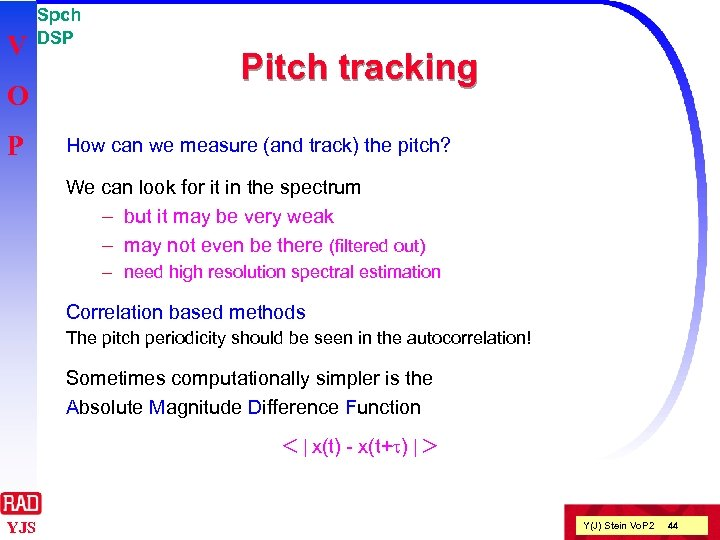V O P Spch DSP Pitch tracking How can we measure (and track) the pitch? We can look for it in the spectrum – but it may be very weak – may not even be there (filtered out) – need high resolution spectral estimation Correlation based methods The pitch periodicity should be seen in the autocorrelation! Sometimes computationally simpler is the Absolute Magnitude Difference Function < | x(t) - x(t+t) | > YJS Y(J) Stein Vo. P 2 44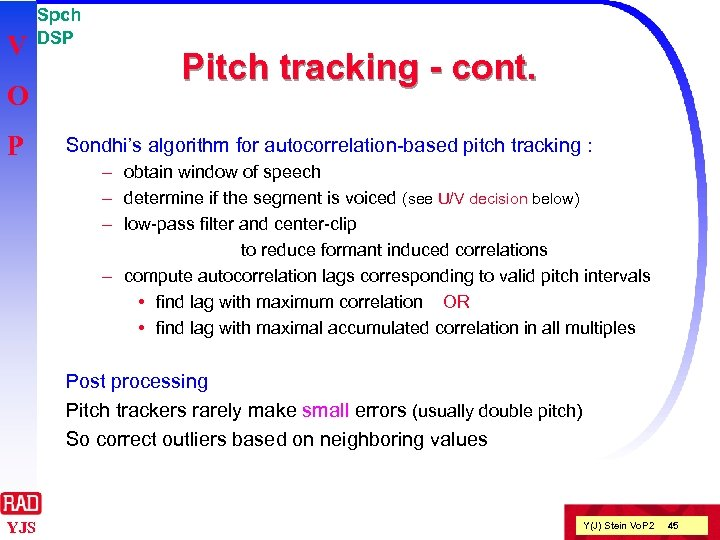V O P Spch DSP Pitch tracking - cont. Sondhi’s algorithm for autocorrelation-based pitch tracking : – obtain window of speech – determine if the segment is voiced (see U/V decision below) – low-pass filter and center-clip to reduce formant induced correlations – compute autocorrelation lags corresponding to valid pitch intervals • find lag with maximum correlation OR • find lag with maximal accumulated correlation in all multiples Post processing Pitch trackers rarely make small errors (usually double pitch) So correct outliers based on neighboring values YJS Y(J) Stein Vo. P 2 45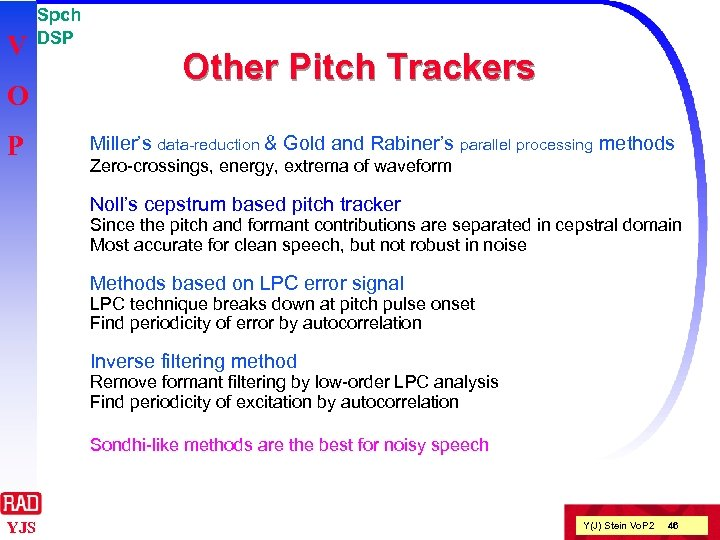V O P Spch DSP Other Pitch Trackers Miller’s data-reduction & Gold and Rabiner’s parallel processing methods Zero-crossings, energy, extrema of waveform Noll’s cepstrum based pitch tracker Since the pitch and formant contributions are separated in cepstral domain Most accurate for clean speech, but not robust in noise Methods based on LPC error signal LPC technique breaks down at pitch pulse onset Find periodicity of error by autocorrelation Inverse filtering method Remove formant filtering by low-order LPC analysis Find periodicity of excitation by autocorrelation Sondhi-like methods are the best for noisy speech YJS Y(J) Stein Vo. P 2 46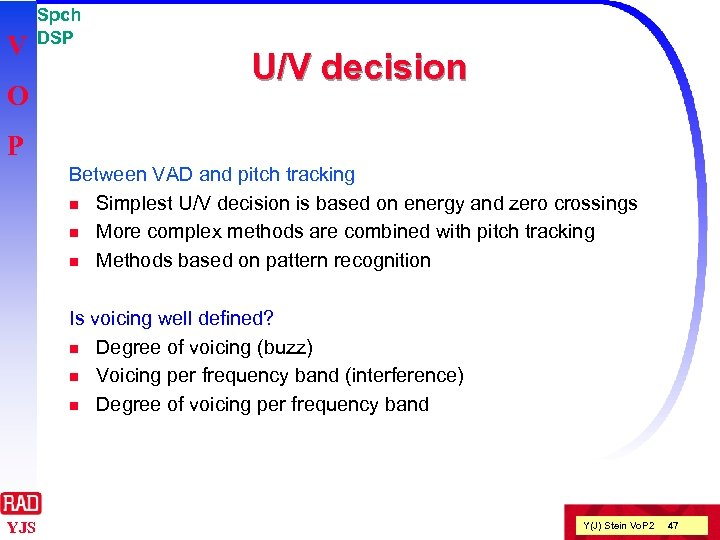V O Spch DSP U/V decision P Between VAD and pitch tracking n Simplest U/V decision is based on energy and zero crossings n More complex methods are combined with pitch tracking n Methods based on pattern recognition Is voicing well defined? n Degree of voicing (buzz) n Voicing per frequency band (interference) n Degree of voicing per frequency band YJS Y(J) Stein Vo. P 2 47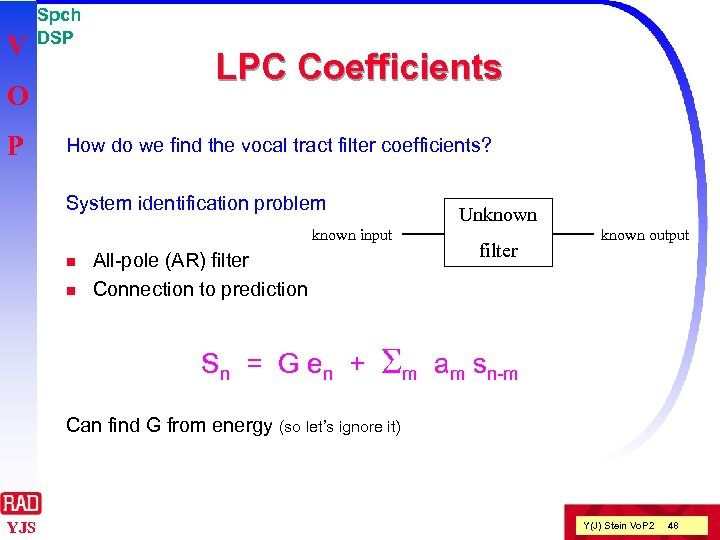V Spch DSP O P LPC Coefficients How do we find the vocal tract filter coefficients? System identification problem Unknown input n n All-pole (AR) filter Connection to prediction Sn = G e n + Sm filter known output am sn-m Can find G from energy (so let’s ignore it) YJS Y(J) Stein Vo. P 2 48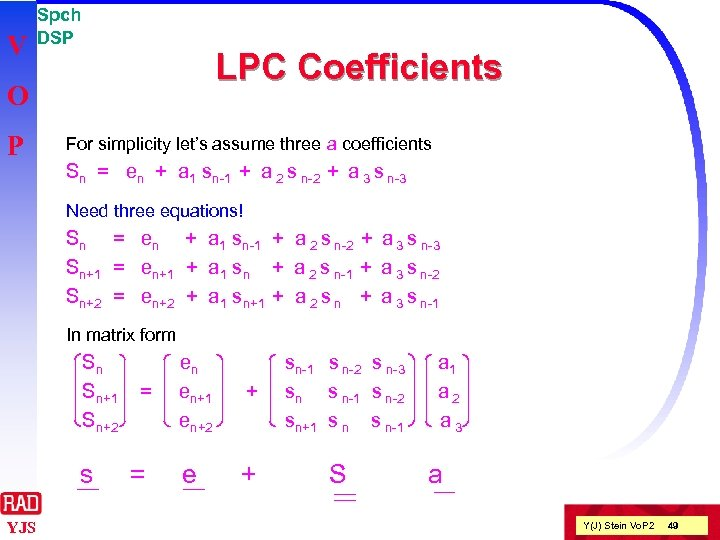V Spch DSP LPC Coefficients O P For simplicity let’s assume three a coefficients Sn = en + a 1 sn-1 + a 2 s n-2 + a 3 s n-3 Need three equations! Sn = en + a 1 sn-1 + a 2 s n-2 + a 3 s n-3 Sn+1 = en+1 + a 1 sn + a 2 s n-1 + a 3 s n-2 Sn+2 = en+2 + a 1 sn+1 + a 2 s n + a 3 s n-1 In matrix form Sn Sn+1 Sn+2 s YJS = = en en+1 en+2 + e + sn-1 s n-2 s n-3 sn s n-1 s n-2 sn+1 s n-1 S a 1 a 2 a 3 a Y(J) Stein Vo. P 2 49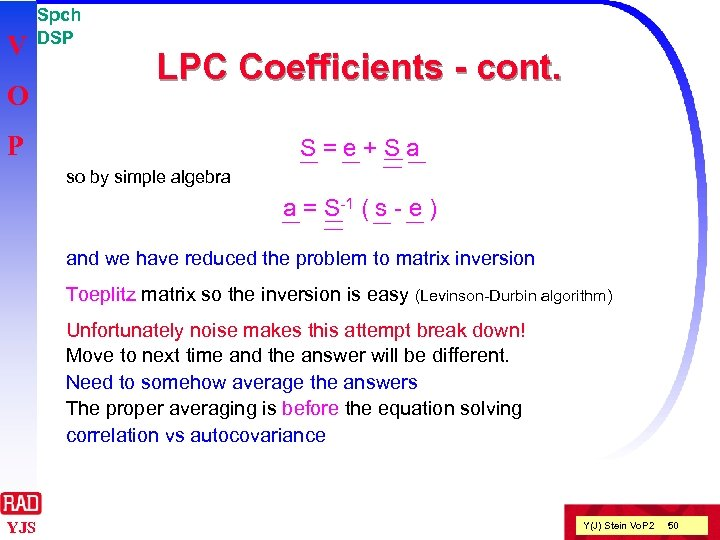V O Spch DSP LPC Coefficients - cont. P S=e+Sa so by simple algebra a = S-1 ( s - e ) and we have reduced the problem to matrix inversion Toeplitz matrix so the inversion is easy (Levinson-Durbin algorithm) Unfortunately noise makes this attempt break down! Move to next time and the answer will be different. Need to somehow average the answers The proper averaging is before the equation solving correlation vs autocovariance YJS Y(J) Stein Vo. P 2 50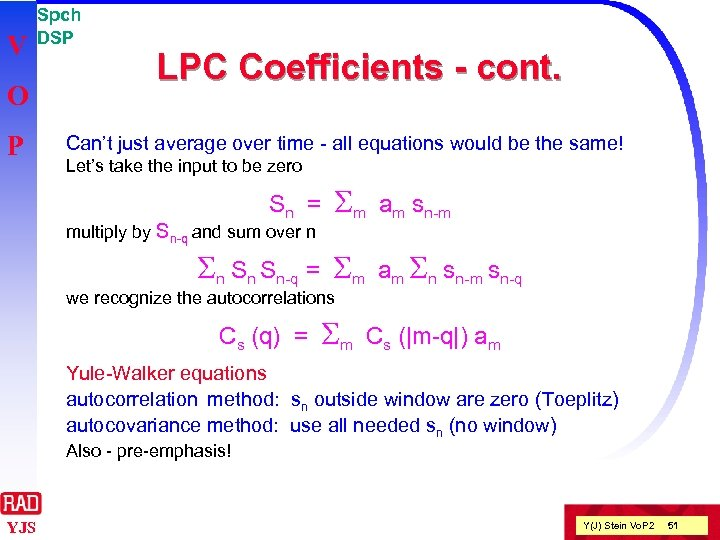V O P Spch DSP LPC Coefficients - cont. Can’t just average over time - all equations would be the same! Let’s take the input to be zero Sn = multiply by Sn-q and sum over n Sm Sn Sn Sn-q = Sm am sn-m am Sn sn-m sn-q we recognize the autocorrelations Cs (q) = Sm Cs (|m-q|) am Yule-Walker equations autocorrelation method: sn outside window are zero (Toeplitz) autocovariance method: use all needed sn (no window) Also - pre-emphasis! YJS Y(J) Stein Vo. P 2 51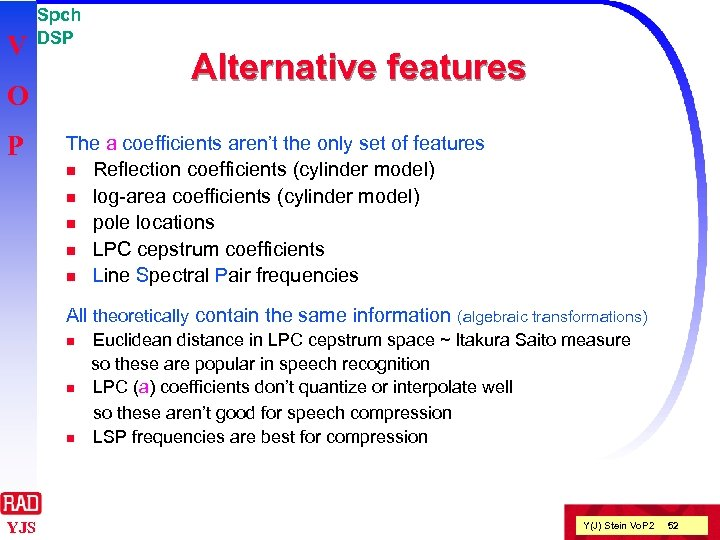V Spch DSP O P Alternative features The a coefficients aren’t the only set of features n Reflection coefficients (cylinder model) n log-area coefficients (cylinder model) n pole locations n LPC cepstrum coefficients n Line Spectral Pair frequencies All theoretically contain the same information (algebraic transformations) n n n YJS Euclidean distance in LPC cepstrum space ~ Itakura Saito measure so these are popular in speech recognition LPC (a) coefficients don’t quantize or interpolate well so these aren’t good for speech compression LSP frequencies are best for compression Y(J) Stein Vo. P 2 52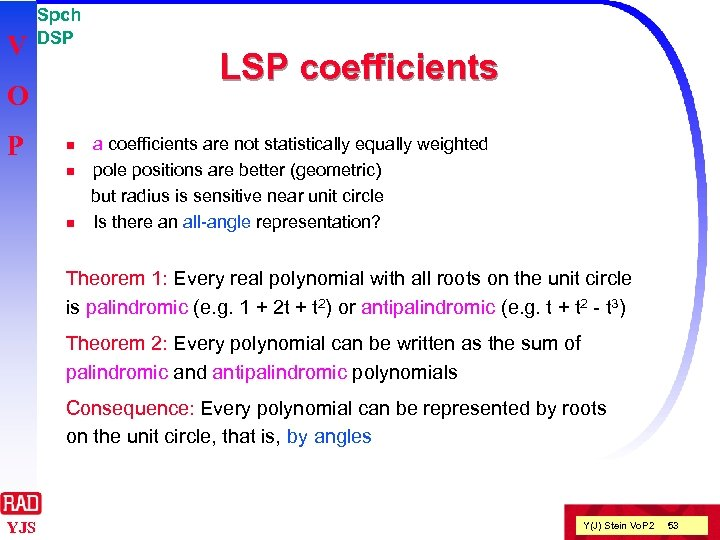V Spch DSP O P n n n LSP coefficients are not statistically equally weighted pole positions are better (geometric) but radius is sensitive near unit circle Is there an all-angle representation? Theorem 1: Every real polynomial with all roots on the unit circle is palindromic (e. g. 1 + 2 t + t 2) or antipalindromic (e. g. t + t 2 - t 3) Theorem 2: Every polynomial can be written as the sum of palindromic and antipalindromic polynomials Consequence: Every polynomial can be represented by roots on the unit circle, that is, by angles YJS Y(J) Stein Vo. P 2 53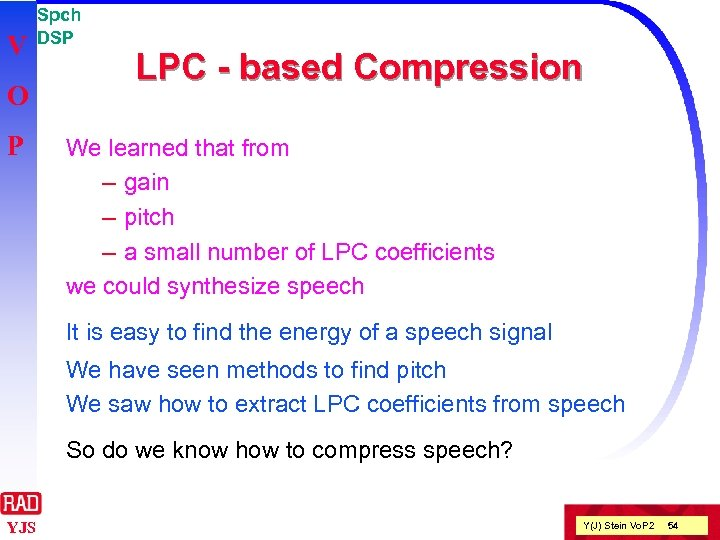V O P Spch DSP LPC - based Compression We learned that from – gain – pitch – a small number of LPC coefficients we could synthesize speech It is easy to find the energy of a speech signal We have seen methods to find pitch We saw how to extract LPC coefficients from speech So do we know how to compress speech? YJS Y(J) Stein Vo. P 2 54